# How a keypad is working? How you can use it in your project?

## Introduction

The keypad is a button matrix which the state (0 or 1) can be detected by a microprocessor such as an Arduino card.

The keypad looks like a matrice, that’s mean each button of a column are linked as an input and each buttons of the same line is linked at a second input.

When we press a button , it’s closing the line and the column of this button and that way the Arduino card knows which button you have pressed.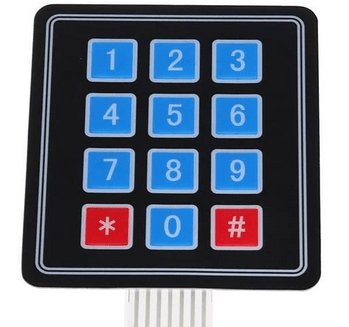The advantage of this matrix is that you can control 16 buttons with only 8 inputs of the Arduino card.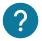### How a keypad is working?

The keypad is divided in two parts: lines and columns.

On each button, there is an open switch on the line which give the LOW state and close the column to have a HIGH state.

If we press one button, the switch will be reversed. That way the Arduino card will know which button has been pressed.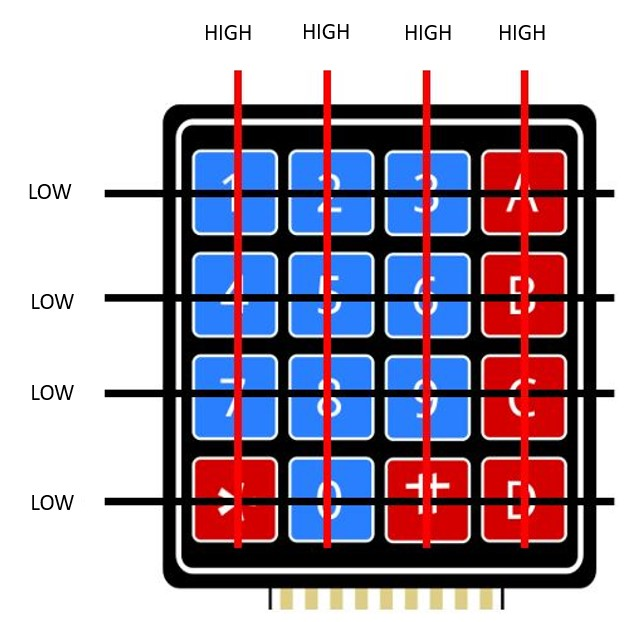This is an example:Here we have pressed the button 5. As we can see the column has a low state and the line at the high state.

The Arduino card just has to meet to both to know that the button 5 has been pressed.

To do a project with the keypad component you will need the keypad library. We will see how to install it.### How to install the keypad library?

The keypad library will be useful for every matrix of button.

Once the library downloaded, you have to open Arduino Ide and click on the sketch/include library and Add .zip library:Then you have to choose the library you have downloaded. At the end you have a notification if the library is correctly installed: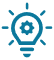If you don’t have a keypad, you can do one on your own with many push button.

We have explained how to do it on the push button course.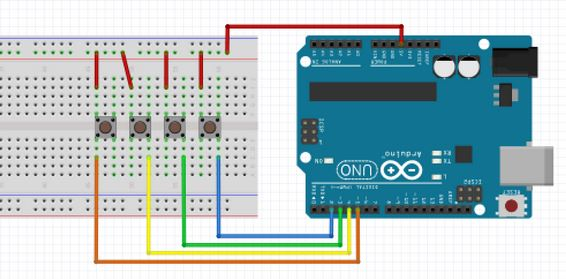## Display the numbers pressed on the serial monitor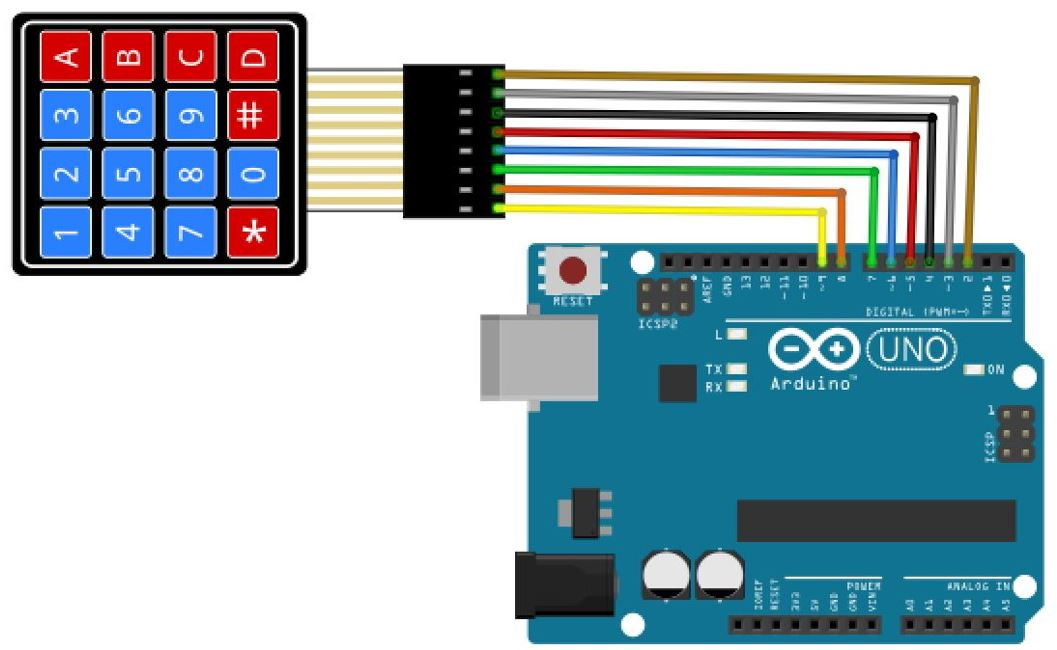You have to compile the program on the Arduino Card:
``````#include <Keypad.h> // Keypad library

const byte Line= 4; // four lines on the keypad
const byte Column = 4; // four columns on the keypad
// We define now the symbols for each buttons.
char hexaButton[Line][Column] = {
{'1','2','3','A'},
{'4','5','6','B'},
{'7','8','9','C'},
{'*','0','#','D'}
};
byte Line_Pins[Line] = {9, 8, 7, 6}; // We connecte the line of the keypad
byte Column_Pins[Column] = {5, 4, 3, 2}; // We connect the column of the keypad

// We initialize the library with the parameters (size of the matrix, symboles)

void setup(){
Serial.begin(9600);// We initialize the serial monitor
}

void loop(){
// We retrieve the number

}
}``````
Here is what you can see on the serial monitor: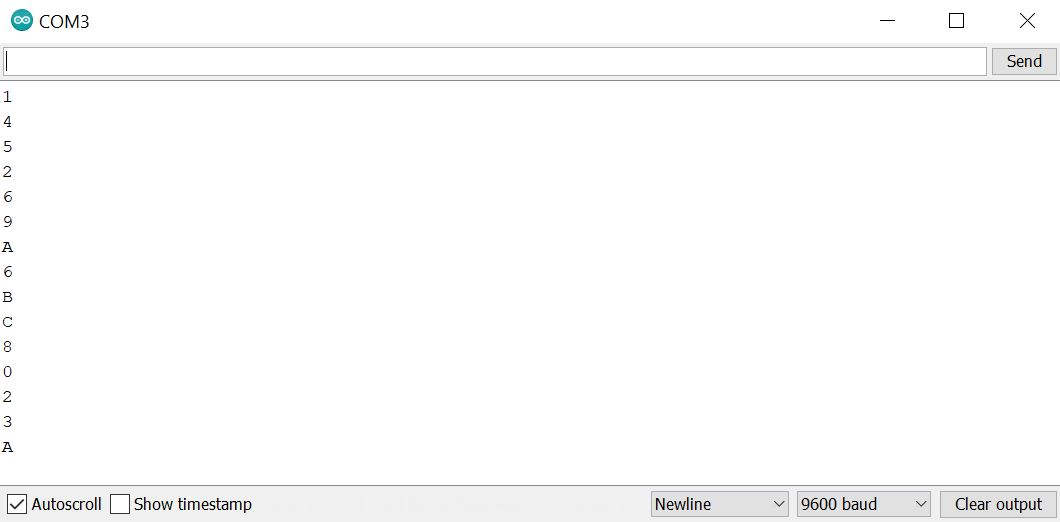## Secret code on the keypad

We will see now how to do a secret code on a keypad. It can be useful for opening a safety deposit box for example.

Here is an explanation of how the program is working:

First the red led is turn on. Then by taping 0123 and D to confirm the secret code, the green led will turn on. If the secret code is wrong and you have pressed on D, the red led will blink.

Finally, if you have pressed the wrong number, you can press the button A to try again.

Here is the sketch of the project: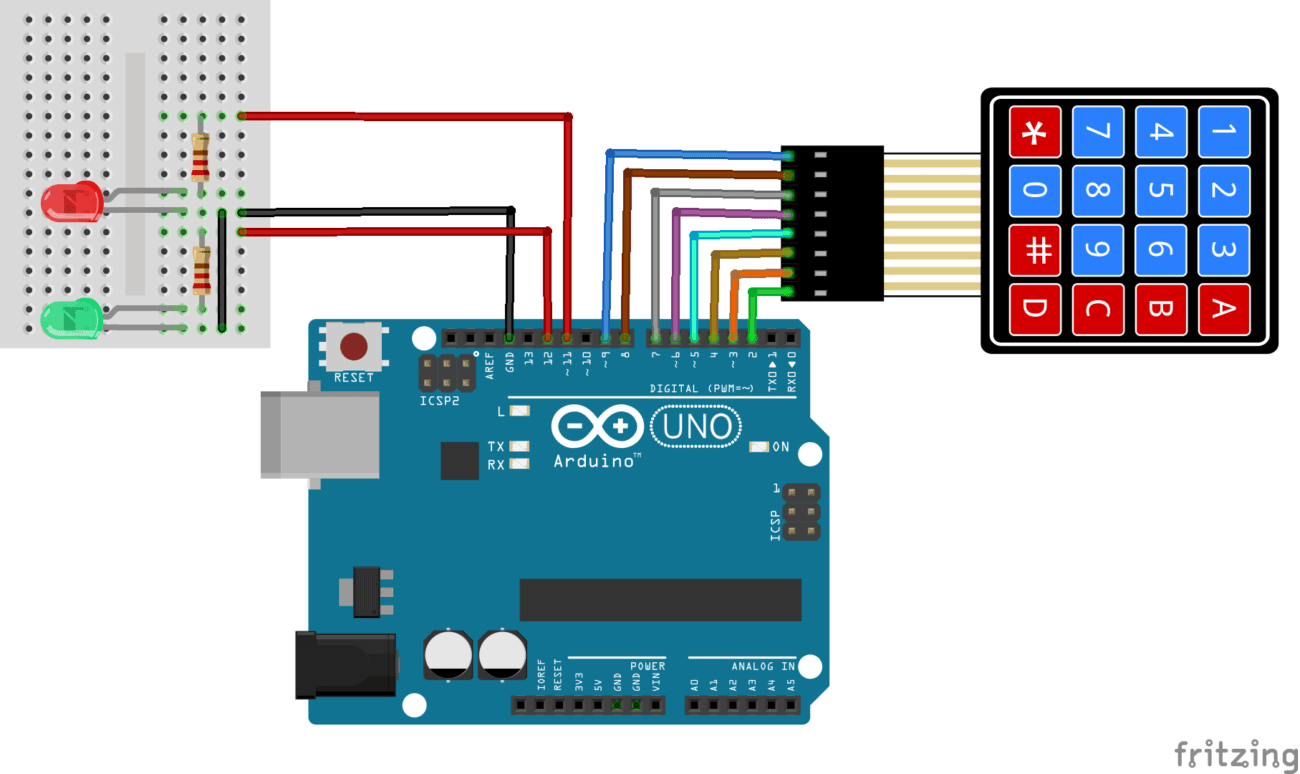Here is the program that you have to compile on your Arduino card:

``````#include <Keypad.h>
int counter =0; // To count the number of button pressed
// We define the two leds
const int red_led = 11;
const int green_led = 12;
const byte Line = 4; // 4 Lines on the keypad
const byte Column = 4; // 4 Columns on the keypad
// We define the symbols for each button
char hexaButton[Line][Column] = {
{'1','2','3','A'},
{'4','5','6','B'},
{'7','8','9','C'},
{'*','0','#','D'}
};
char code; // Contains the buttons pressed by the user
byte Line_Pins[Line] = {9, 8, 7, 6}; // Connect the line of the keypad
byte Column_Pins[Column] = {5, 4, 3, 2}; // Connect the column of the keypad

// Initiliaze the library with our parameters (size of the matrix and symbols)

void setup(){
pinMode(red_led,OUTPUT); // We assign the red led as an output
pinMode(green_led,OUTPUT); // We assign the green led as an output
digitalWrite(red_led, HIGH); //We turn on the red led at the beginning because by default the safety box is closed
}

void loop(){
if (keypad_matrix){ // If a button has been pushed
code[counter] =keypad_matrix; // We put the value on the array
counter+=1; // We increments the counter
if (keypad_matrix =='A'){// If the user push the A button, it reset the values
counter=0;
}
if (counter ==5){ //  If we have 4 valuesn we verify if the password is correct. If not, we reset the counter.
if (code =='0' && code =='1' && code =='2' && code =='3' && code =='D'){ // If the code is correct
digitalWrite(red_led, LOW); // Turn off the red led
digitalWrite(green_led, HIGH); // turn on the green led Allume la led verte
}

if (code !='0' || code !='1' || code !='2' || code !='3' && code =='D'){ // If the code is wrong
digitalWrite(green_led, LOW); // Turn off the green led if it was turn on